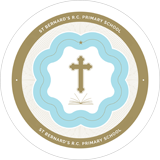# St. Bernard's RC Primary

••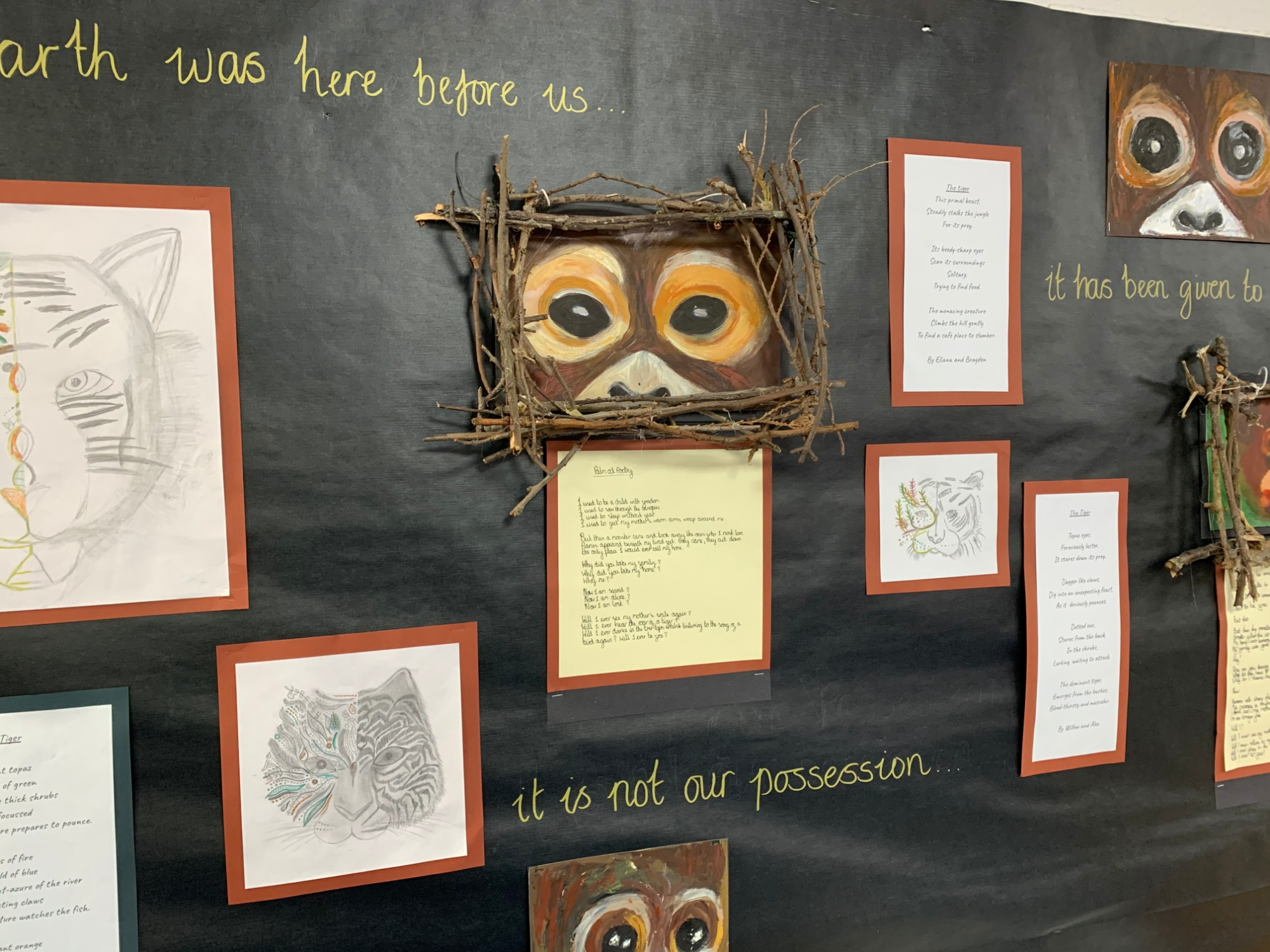•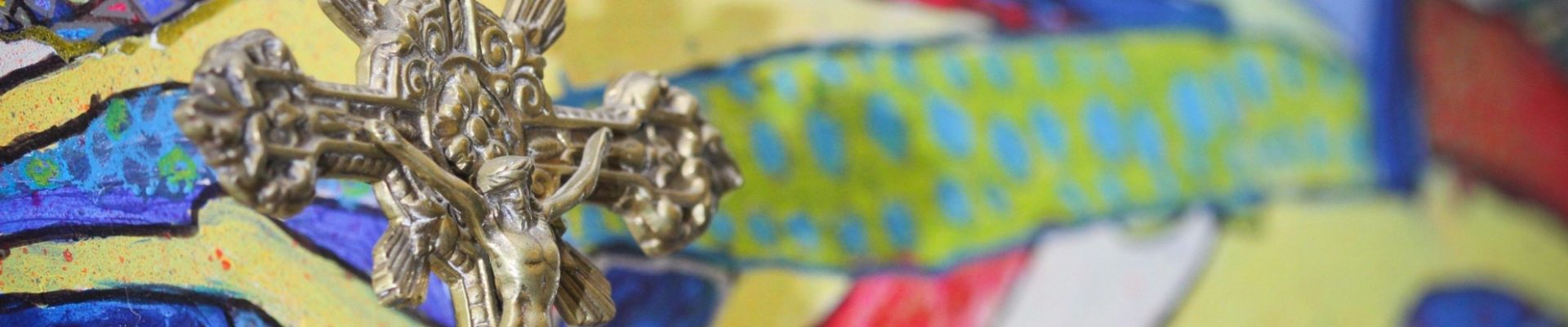•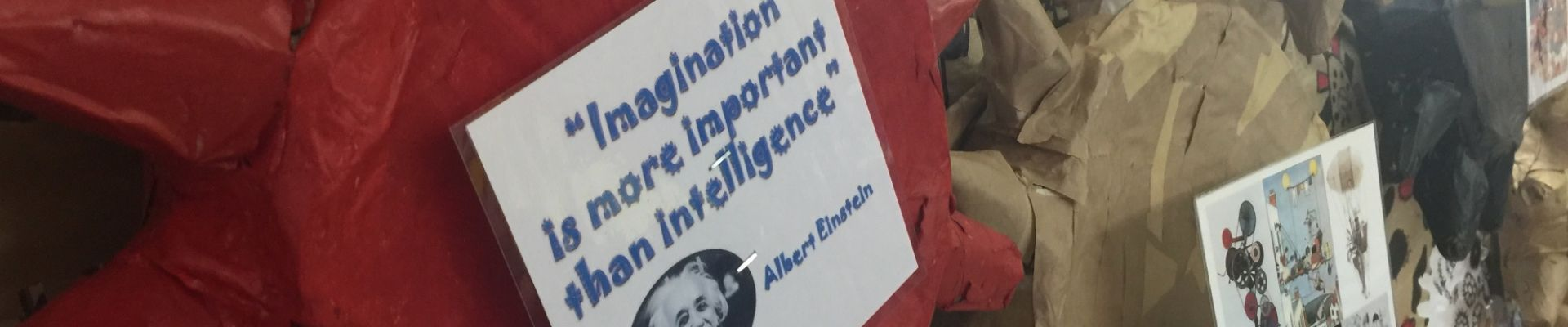••••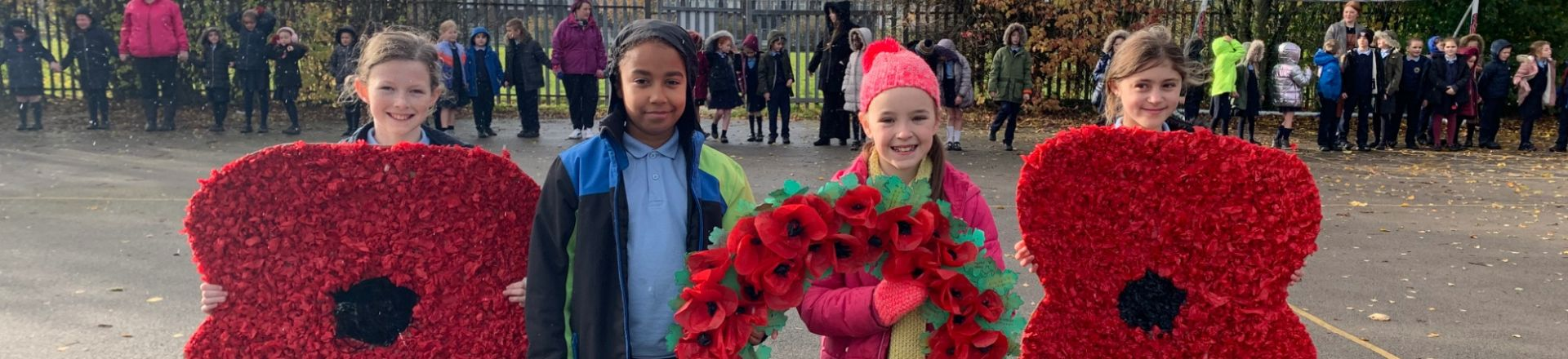# Maths No problem

Here at St Bernard's we use Maths No Problem to enable children to master maths skills.

It is based on the research and lesson approaches used in Singapore.

Here is some information on how a maths lesson is structured.

Maths Lesson Structure

It is important that the children work with concrete objects first to help develop their understanding. They can then move onto pictorial representations and finally the abstract- writing the numbers. This video provides more information about the CPA approach.

Using the CPA approach in different mathematical concepts.

Maths Year 1

Maths Year 2

Maths Year 3

Maths Year 4

Maths Year 5

Want to find out more about the different mathematical methods? Visit http://www.mathsnoproblem.co.uk/parent-videos